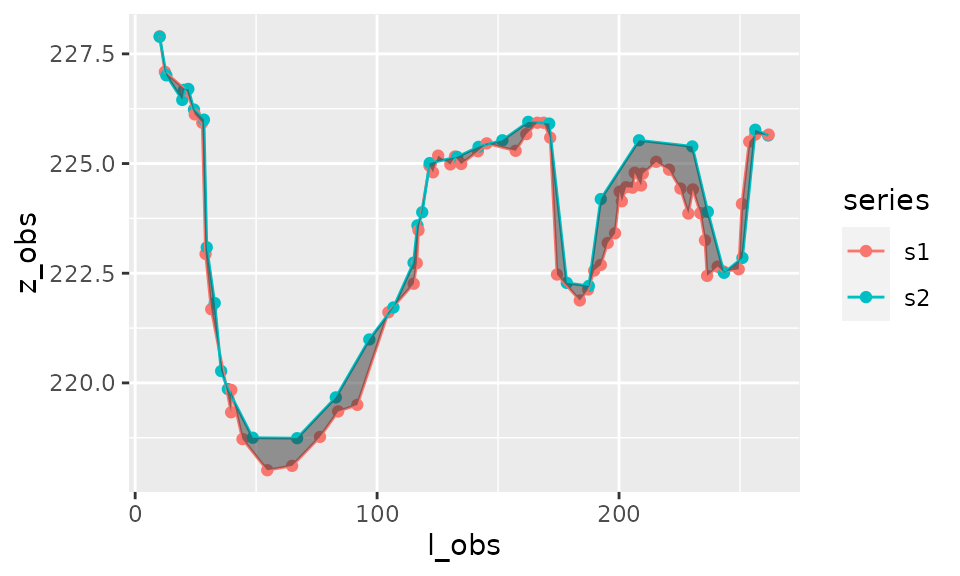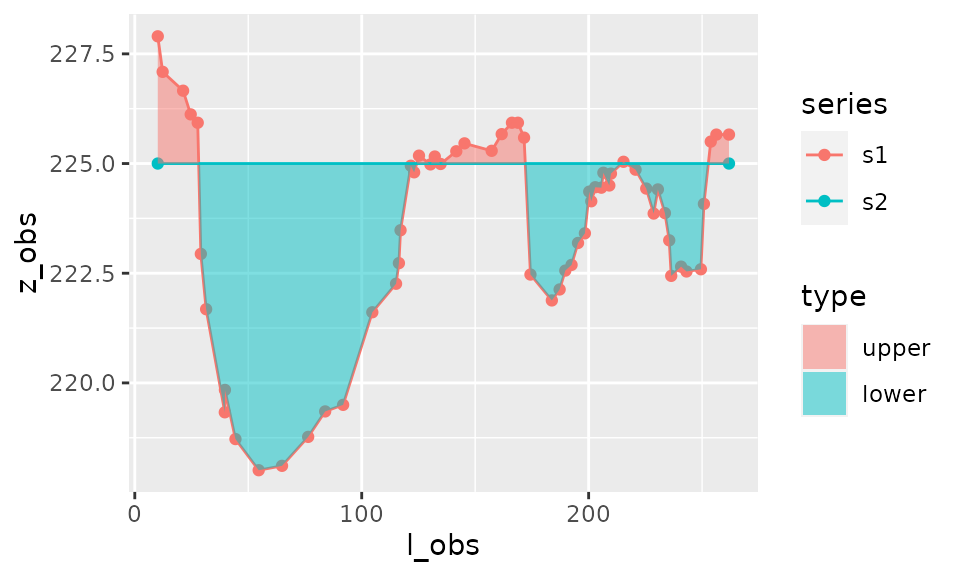library(riverbed)
library(dplyr)
library(ggplot2)

# Example datasets

data(s1)
data(s2)

We consider two longitudinal series $$s_1=(l_1,z_1)$$, and $$s_2=(l_2,z_2)$$:# Principle of the area calculation

To calculate the area between the curves we need to interpolate the data and calculate intersects between the two curves:# Use of area_between()

This, as well as the calculation of corresponding trapezia, is realised by the function area_between().

result_area <- area_between(s1,s2)
result_area
#> $data #> # A tibble: 237 x 13 #> l z1 p z2 a type l_obs z_obs series zmin zmax lmin #> <dbl> <dbl> <chr> <dbl> <dbl> <fct> <dbl> <dbl> <chr> <dbl> <dbl> <dbl> #> 1 10.1 228. observ… 228. 0 <NA> 10.1 228. s1 NA NA NA #> 2 10.1 228. observ… 228. 8.68e-4 upper 10.1 228. s2 NA NA NA #> 3 10.3 228. inters… 228. NA upper NA NA <NA> NA NA NA #> 4 10.3 228. inters… 228. -1.13e-1 lower NA NA <NA> NA NA NA #> 5 12.3 227. observ… 227. 0 <NA> 12.3 227. s1 NA NA NA #> 6 12.3 227. interp… 227. -2.40e-2 lower NA NA s2 NA NA NA #> 7 12.7 227. inters… 227. NA lower NA NA <NA> NA NA NA #> 8 12.7 227. inters… 227. 4.79e-3 upper NA NA <NA> NA NA NA #> 9 12.9 227. interp… 227. 0 <NA> NA NA s1 NA NA NA #> 10 12.9 227. observ… 227. 1.14e+0 upper 12.9 227. s2 NA NA NA #> # … with 227 more rows, and 1 more variable: lmax <dbl> #> #>$area
#>  -98.07248
#>
#> $area_by_type #> # A tibble: 2 x 2 #> type area #> <fct> <dbl> #> 1 upper 7.76 #> 2 lower -106. #> #>$sigma_area
#>  0

The output of the function corresponds to

• total area between curves (i.e. positive area - negative area)
• area between curves according to whether first curve is above the second curve (“upper”) or below (“lower”)
• data with all coordinates of interpolated points and intersects, used to calculate the area of trapezia (and which will be used by function plot_area())

Note that it is also possible to calculate the area between one longitudinal series $$s_1=(l_1,z_1)$$ and a constant horizontal value $$h$$.

result_area_h <- area_between(s1,h=225)

# Plot of area

plot_area(result_area)If the distinction between upper and lower area is irrelevant then it is possible not to distinguish these two types on the plot:

plot_area(result_area,distinguish_type=FALSE)The plotting function plot_area() can also be used with just one series and constant horizontal level $$h$$, as calculated earlier:

plot_area(result_area_h)